Subsections

# 3.4 Methods of data processing

The first step of the data processing is to convert the raw (logged) data into a time series according to a particular averaging or summing period. The second step is to use these time series for further calculations. Conversion and calculation methods are summarised in this section.

The average of a finite sequence of samples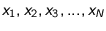is commonly defined as: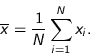(3.1)

The standard deviation of a finite sequence of samples is defined as: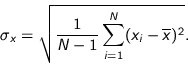(3.2)

The standard deviation can also be calculated from the sum of the squared sample values (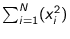):(3.3)

The coefficient of determination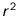, or correlation coefficient, of estimated values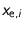in relation to their measured values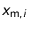is calculated by eq. 3.4 [Montgomery and Runger 1999, p. 464].(3.4)

The averaging or summation period is denoted by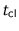, and called clock period'. The start and end of each period are synchronised with clock and calendar. The first clock period of a day starts at 0h00.

## 3.4.1 Reference wind data

The output of the ultrasonic anemometers is directly given in a standard unit of velocity, for every component (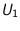,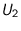,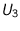) (figure 3.10). One-minute averages and sums of squared output values of the anemometer on the mast of the Auditorium are calculated and form the raw data. For a chosen period, the mean wind speed components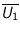,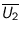and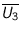are calculated from the one-minute data.

Next, these mean wind speed components are transformed into the global axis system (figure 2.4):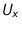,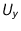,. Furthermore, the quantities,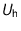,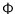,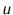,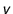and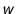can be calculated with the definitions given in section 2.1.5.

The raw data of the ultrasonic anemometer mounted on the boom at façade position P4 consists of 1 second values (,,) (figure 3.10). From these, the-averaged quantities,,,,andare calculated.

## 3.4.3 Horizontal rain data

Horizontal rain data are obtained by three devices, two tipping-bucket rain gauges and a rain indicator. The horizontal rain intensity during a clock periodis calculated from the number of tippings during this period: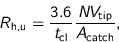(3.5)

with= the (uncorrected) horizontal rain intensity [mm h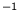],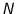= the number of tips during[s],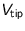= the effective volume [ml] of a bucket, and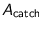= the catchment area [m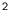] of the rain gauge.

In fact the horizontal rain intensityis an average during.

If the sample rate of the rain indicator is denoted by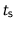, the rain duration during a clock periodis calculated by: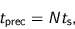(3.6)

with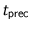= the rain or precipitation duration [s] during, and= the number of samples at which the rain indicator senses rain or other forms of precipitation during the clock period.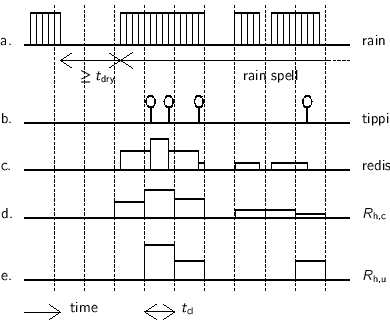Corrected horizontal rain intensityThe data of the rain indicator and one of the rain gauges can be combined to correct rain intensity data, especially during shorter averaging periods. A method is presented in this section. The upper two graphs of figure 3.20 are sketches of rain indicator and rain gauge readings as a function of time. The rain amount registered by the first tipping in a period, could have been collected during previous clock periods. The reading of the rain indicator can be of help to estimate how to redistribute' this amount over the previous periods. To prevent that rain is distributed over too many previous periods, a minimum time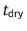is defined. This minimum time divides time into dry periods and periods with more or less continuous rain (i.e. rain spells). The result of the redistribution of rain amounts of the registered tippings is sketched in figure 3.20c. The redistribution is done in proportion to the precipitation times measured by the rain indicator. Summing up the redistributed rain amounts per clock periodyields the so-called corrected rain intensity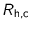(figure 3.20d). For comparison, the uncorrected rain intensitycalculated with eq. 3.5 is sketched in figure 3.20e.

## 3.4.4 Raindrop spectrum data

Every 30 s, the disdrometer reports the mean particle velocity in every size class and the particle number concentration in every size class. We will denote these quantities with[m s] and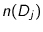[m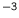m], respectively. The raindrop mass flux per drop size class per 30 s (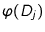[kg m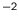(30 s)]) can be calculated from these two quantities by (cf. eq. 2.15 and 2.16):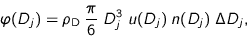(3.7)

with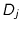and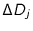= the centre and interval of the drop size class in m (table 3.4), and= density [kg m] of water.

The reported spectra are only taken into account if the disdrometer indicates that the precipitation is drizzle or rain. So, spectra of snow, hail and mixtures are discarded.

The disdrometer output has two disadvantages for our purposes. Firstly, the disdrometer gives the raindrop number concentration spectrum, which is calculated from the quantity which we are interested in, namely the raindrop mass flux spectrum. We have to `recalculate' the raindrop mass flux spectrum by use of the given averaged particle velocities. Secondly, the interval of the size classes increases with the centre diameter, which results in larger absolute errors of the real mass flux at larger diameters. The two disadvantages can be solved by outputting every detected raindrop with its diameter and velocity, but it was not possible to us to adapt the software in the data processing unit of the disdrometer.

## 3.4.5 Driving rain data of the TUE-I and TUE-II gauges

In gauges TUE-I and TUE-II, the driving rain collected in a reservoir is measured by a balance, of which simply the weight values are logged. The (mean) driving rain intensity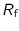during a clock periodis calculated from the difference between begin and end values of the logged mass: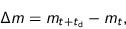(3.8)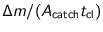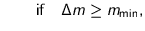(3.9)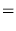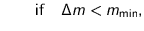with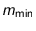= a threshold value [kg] for the minimum detectable mass difference during a clock period,= the catchment area [m] of the driving rain gauge, and= the clock period in h.

By the threshold valueone suppresses variations of the measured mass value due to e.g. temperature changes. It depends on the resolution of the balance (= 0.105 g was found suitable for the applied balances with a resolution of 0.1 g).

## 3.4.6 Driving rain data of the CTH and DTU gauges

The number of tippings of the CTH driving rain gauge is logged two times per second. The driving rain intensitycan be calculated similar to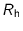, eq. 3.5.

The raw data of the DTU gauge consists of voltage values of the strain gauge. The data processing method was already mentioned in section 3.2.6. The resulting driving rain intensity is calculated from the difference of the 10-min averaged masses, of two subsequent 10-min clock periods, similar to eq. 3.9. Driving rain intensities for larger clock periods are calculated from the obtained 10-min driving rain intensities.

© 2002 Fabien J.R. van Mook
ISBN 90-6814-569-X
Published as issue 69 in the Bouwstenen series of the Faculty of Architecture, Planning and Building of the Eindhoven University of Technology.